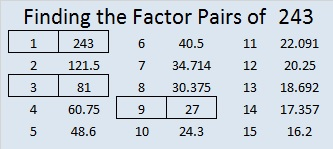# 243 and Level 5

• 243 is a composite number.
• Prime factorization: 243 = 3 x 3 x 3 x 3 x 3, which can be written 243 = (3^5)
• The exponent in the prime factorization is 5. Adding one we get (5 + 1) = 6. Therefore 243 has 6 factors.
• Factors of 243: 1, 3, 9, 27, 81, 243
• Factor pairs: 243 = 1 x 243, 3 x 81, or 9 x 27
• Taking the factor pair with the largest square number factor, we get √243 = (√3)(√81) = 9√3 ≈ 15.588This puzzle is for my sister, who is great at completing these Level 5 puzzles. Here is a black and white version for those who may prefer it:Print the puzzles or type the factors on this excel file: 12 Factors 2014-09-221.Joseph Nebus